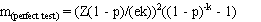# 12 - Sample size for fixed pool size and perfect test

This program calculates the approximate numbers of pools required for a range of pool sizes and specified values for estimated prevalence and desired confidence and precision of the estimate, assuming fixed pool sizes and a test with 100% sensitivity and specificity. See Worlund & Taylor (1983) for more details.

The required number of pools (m) to estimate the true prevalence with the desired precision is calculated as:where:

• p = assumed true prevalence;
• k = pool size;
• e = the acceptable error (desired precision); and
• Z = the standardised normal variate corresponding to the desired level of confidence.

For fixed pool size and perfect tests, the optimum value of m can be calculated that minimises the variance of the estimated prevalence and consequently minimises the number of pools requiring testing to achieve the desired confidence and precision. This optimum value for m depends on the prevalence and is approximately 1.6/pi. This equates to the pool size which results in an expected number of 1.6 infected individuals per pool. See Sacks et al. (1989) for more details. Prevalence estimates may be upwardly biased, particularly as the probability of all pools testing positive increases (high prevalence and/or small numbers of large pools). Therefore, it is advisable to select a lower value for pool size and test a larger number of smaller pools to minimise potential bias in the result.

Required inputs for this analysis are:

• the assumed true prevalence;
• the desired level of precision (or acceptable error); and
• the desired level of confidence in the result.

For example, you might wish to estimate the prevalence where the true value is assumed to be about 0.01 (1%), and you wish to have 95% (0.95) confidence that the true value is within +/- 0.005 (0.5%) of your estimate. The assumed prevalence, desired precision and level of confidence must all be >0 and <1.

You can also input a suggested pool size if desired, and the program will calculate the corresponding number of pools to be tested for that pool size (in addition to predetermined pool sizes). Suggested pool size is ignored if it is zero.

Output from the analysis is:

• the number of pools required for the input-scenario and the suggested pool size;
• the number of pools required for the input-scenario and the optimum pool size;
• a table of the numbers of pools (and total number of samples) required for the input-scenario for various pool sizes ranging from 1 to 500; and
• a graph of number of pools vs pool size.

Contents
1 Introduction
2 Overview
3 Bayesian vs Frequentist methods
4 Fixed pool size and perfect tests
5 Fixed pool size and known Se & Sp
6 Fixed pool size and uncertain Se & Sp
7 Variable pool size and perfect tests
8 Pooled prevalence using a Gibbs sampler
9 True prevalence using one test
10 Estimated true prevalence using two tests with a Gibbs sampler
11 Estimation of parameters for prior Beta distributions
12 Sample size for fixed pool size and perfect test
13 Sample size for fixed pool size and known test sensitivity and specificity
14 Sample size for fixed pool size and uncertain test sensitivity and specificity
15 Simulate sampling for fixed pool size
16 Simulate sampling for variable pool sizes
17 Important Assumptions
18 Pooled prevalence estimates are biased!